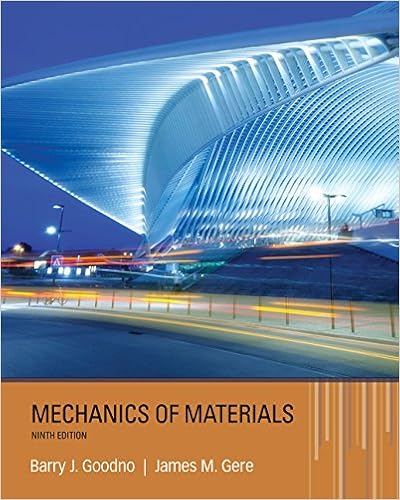# Solution a rate in 005 2 0001 rate out 2 q t 200 001

• Test Prep
• 7
• 100% (3) 3 out of 3 people found this document helpful

This preview shows page 5 - 7 out of 7 pages.

##### We have textbook solutions for you!
The document you are viewing contains questions related to this textbook.The document you are viewing contains questions related to this textbook.
Chapter 8 / Exercise 8.2-3
Mechanics of Materials
Gere/GoodnoExpert Verified
Solution
t).
.
> 0.
##### We have textbook solutions for you!
The document you are viewing contains questions related to this textbook.The document you are viewing contains questions related to this textbook.
Chapter 8 / Exercise 8.2-3
Mechanics of Materials
Gere/GoodnoExpert Verified
MAT1322-3X Solution to Final Examination Summer 2017 6
0.
. 4. (6 marks) Use an appropriate test method to determine whether each of the following series is convergent or divergent. (a) 21(ln)nnn; (b) 221nnnn. 3
n
2
3
3
MAT1322-3X Solution to Final Examination Summer 2017
6. (4 marks)Suppose a function z= f(x, y) is defined implicitly by the equation F(x, y, zwhere F(x, y, z) = x3y2z+ xyz(a) (1 mark) Find the partial derivative zxand zyat the point (1, 2, −1).(b) (1 mark) Find the equation of the tangent plane of the graph of this equation at the point (1, 2, −1).(c) (1 mark) Find the directional derivative of this function at point (1, 2, −1) in the direction of the vector u= 34,55) = 3, 3. .
•••